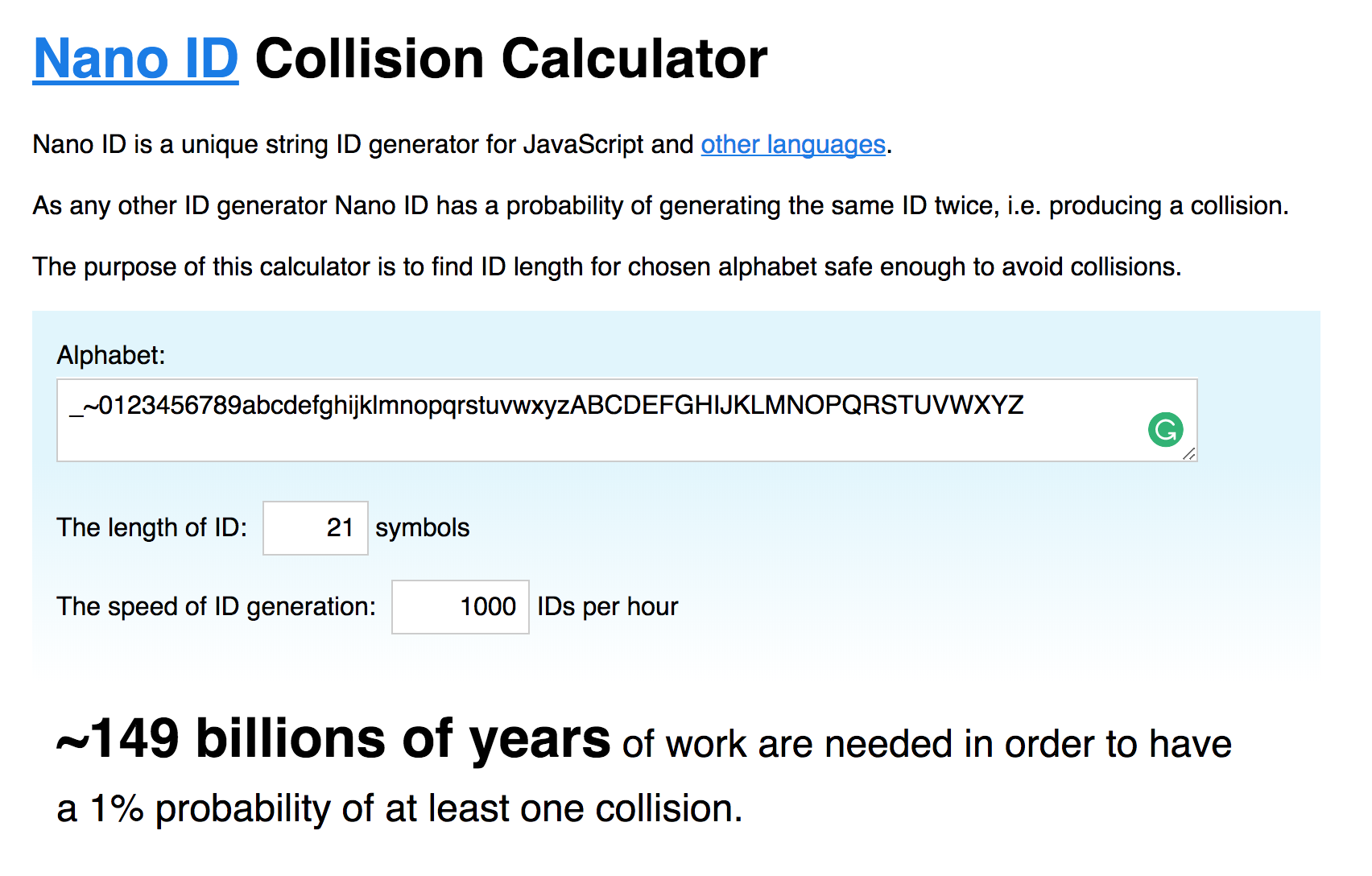# What is Random

2216 days ago, 0 views.

random is a term used to describe the process of generate data with no correlation.

Random number generation is used around many users cases over computers such as:

• Pick a random item from an array.
• Generate unique keys from an API.
• Create the key exchange between an HTTPS connection.

As you can see, all these examples use a random factor, but they are different. Also computationally they are different so, how to?

Like most of the things in life, it depends.

## Types of “random”

We can distinguish at least three types of random data generators. These depends on our use case: Generally a high entropy process take more time (or 💰), but not always you need it.

### Truly Random

The ideal case. True randomness, to which no pattern or algorithm applies. It’s debatable whether this really exists.

To have any hope of producing truly random data, you must reach outside the computer and sample the analog world. This means use specific hardware for this purpose as well.

At Cloudflare they use a wall of lava light to generate enough entropy to encrypt the requests.

### Pseudo Random

Also known as Pseudo-Random Number Generators (PRNGs).

As the name suggest, is very close to truly random numbers generators, but the output is generated using a finite set of numbers:

• The current process ID.
• The tick count since boot time.
• How many time takes an `npm install`.

They are deterministic because they use an algorithm or a mathematical formulae for be calculated but, on the other hand, they are sufficiently random for practical purposes and they make the process more efficient.

`Math.random` is an example of a way to get a pseudo-random number between `0` and `1`.

In cryptographically terms, `Math.random` doest not provide a secure random number. This means, probably you can use it to pick a random element from an array, but because the implementation is not robust enough, it doesn’t have the characteristics to be considered cryptographically secured.

A subsection inside PRNGs are Cryptographically Secure Pseudo-Random Number Generator (CSPRNG).

Every random value that you need for security-related purposes (ie. anything where the possibility of an “attack” exists), should be generated using a CSPRNG. This includes:

• Lottery numbers.
• API keys.

Theses examples are very close with nonce concept.

## From the code side

In Node.js, the most widely available CSPRNG is the crypto.randomBytes:

``````const crypto = require('crypto')
const {promisify} = require('util')

const randomBytes = promisify(crypto.randomBytes)

;(async () => {
const buffer = await randomBytes(256)
console.log(`\${buffer.length} bytes of random data`)
console.log(buffer.toString('hex'))
})()
``````

The code above generates raw pseudo random data.

You need to be careful transforming the data because wrong data manipulation will modify how random it is.

If you need specific random output for your user case, I recommend you use the following libraries:

• random-number-csprng – Get a secure random number between a range.
• uuid – Universally unique identifier, supporting multiple versions.
• nanoid – A tiny, secure URL-friendly unique string ID generator for JavaScript.The world is random. Computers aren’t.

## BibliographyKiko Beats